Smartick is a fun way to learn math!Jun09

# Properties of Multiplication

#### The properties of multiplication are distributive, commutative, associative, removing a common factor and the neutral element.

We are dedicating this post to examining the properties of multiplication, which are the following:

• Distributive property: The multiplication of a number by a sum is equal to the sum of the multiplications of this number by each one of the amounts to be added.

Lets take for example: 2 x (3 + 5)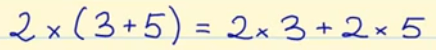According to the distributive property 2 x (3 + 5) will be equal to 2 x 3 + 2 x 5.

Lets check if this is true.

2 x (3 + 5) = 2 x 8 = 16

2 x 3 + 2 x 5 = 6 + 10 = 16

Both give us 16 as a result, which shows that the distributive property of multiplication works.

• Commutative property: The order of the factors does not change the product.

Let’s look at an example of the commutative property: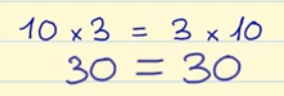The result of multiplying 10 x 3 will be equal to multiplying 3 x 10. Although we change the order of the factors, the result is still 30.

• Associative property: The mode of grouping the factors does not change the result of the multiplication.

Let’s take an example of the associative property of multiplication: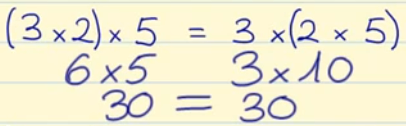In this case, as we show in the image, it gives us the same result if we multiply 3 x 2 and then multiply the result by 5 as if we multiply 2 x 5 and then multiply the result by 3.

• Removing a common factor: This is the inverse property of the distributive property. If various addends have a common factor, we can transform the sum into a product by taking out this factor.

Let’s look at an example of removing a common factor. If we have the operation (2 x 7) + (3 x 7), which has 7 as a common factor, we can transform this operation into 7 x (2 + 3).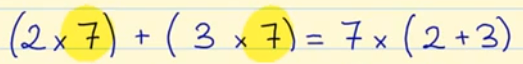Let’s check that removing the common factor gives us the same result:

(2 x 7) + (3 x 7) = 14 + 21 = 35

7 x (2 + 3) = 7 x 5 = 35

This shows that this property of multiplication works.

• Neutral Element: 1 is called the identity of multiplication because every number multiplied by itself is just the same number.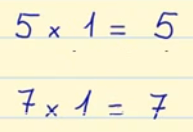In the example that we show in the image, we see that if we multiply 5 or 7 by 1, we get as a result 5 or 7. So any number that we multiply by 1 gives us, as a result, the same number.

Fun is our brain’s favorite way of learning
Diane Ackerman
Smartick is a fun way to learn math
• 15 fun minutes a day
• Millions of students since 2009• girmaDec 11 2021, 2:37 PM

Excelent reference.

• AlexDec 04 2021, 2:55 PM

I couldn’t remember the properties of multiplication. This was helpful for my homework.

• jackNov 07 2021, 11:51 AM

how can i login to this ???????????????????

• Smartick HelpNov 08 2021, 3:49 AM

Hello Jack:

In order to see if Smartick is the right fit for your child, why not have them give it a try? Smartick offers a trial absolutely free of charge and without any required commitment to continue after. Your children can use it daily to work on and develop their math skills. If you would like to continue working with us after the trial period, you have the option to subscribe. Otherwise, your account will simply be deactivated.

If you are using a computer, you can start your free Smartick trial by registering through the following steps:
1. Go to our webpage and click on Register Free
3. Click “Add another student” to register more students
5. Have your child begin their first session!
Just to clarify, Smartick is not optimized to be used through mobile phones. We instead recommend using a computer (Windows, Linux or Mac) through browsers Google Chrome or Firefox, or a tablet (recommended from 9 inches on) through the Smartick app that’s available for both Android (from 4.1) and iOS (from iOS 7.0). You can download the Smartick app for iPad from the Apple Store, and for Android tablets from the Play Store. If you prefer using a tablet, we recommend always using the Smartick app to get the best user experience.

If you have any other questions or need further assistance, please let us know! We’re always happy to help.
Our communication channel for any questions you may have is our e-mail: [email protected]
Best,

• MarivicJun 15 2021, 4:42 AM

It is easy to comprehend we need more of the work sheets

• BriOct 29 2020, 1:04 PM

I thought there were four multiplication properties. Hmm… nope. Only three that I can think of. Great job guys! This is very helpful! I’m doing a math paper and I couldn’t remember the multiplication properties. Thanks!

• Ashutosh jaiswalAug 07 2020, 6:09 AM

Sir, it is very useful for all the students.